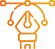### Sun Sight and Star Sight

During navigation an observation of the sextant altitude of the sun during the day or a single observation of sextant altitude of a star during evening twilight or morning twilight may be required to obtain the elements of Astronomical position line True Bg. and the Intercept As it is known, the astronomical observations consist of time (UT) and the sextant altitude

The following figure shows the solution sequence for obtaining the astronomical position line elements on the basis of which the program was designed

Apply it now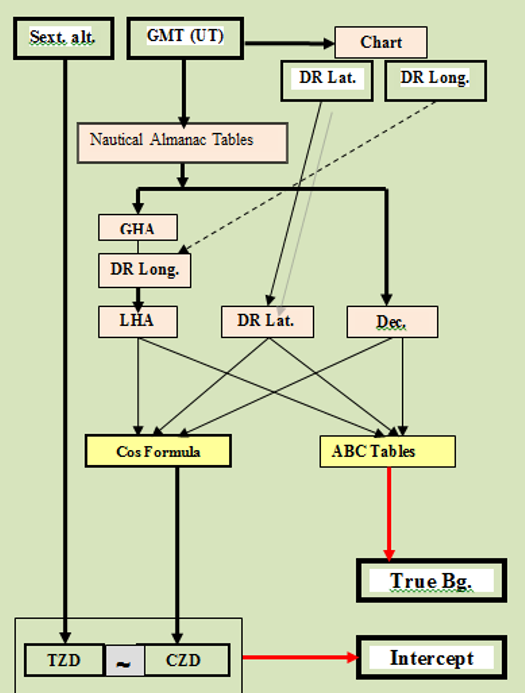### Fixed Position from Two Sun Sights

During the day it is not available to observe any celestial body except the sun, of course Therefore, determining the observed position requires two observations of the sun between them a relatively long sailing period (determined by the change in the true bearing of the sun by about 30 at least) Then the first position line is shifted to the time of the second position line where the point of their intersection is the observed location at the time of the second observation The figure shows this visualization to solve this problem..

The program has been designed as follows:

• Solving the 1st sun sight using 1st DR position to obtain the elements of the 1st PL.
• Calculating the sailing parameters between the two observations and obtaining the 2nd DR position.
• Solving the 2nd sun sight using 2nd DR position to obtain the elements of the 2nd PL.
• Make run of the 1st PL to the time of the 2nd PL; the point of intersection is the fixed position.
Apply it now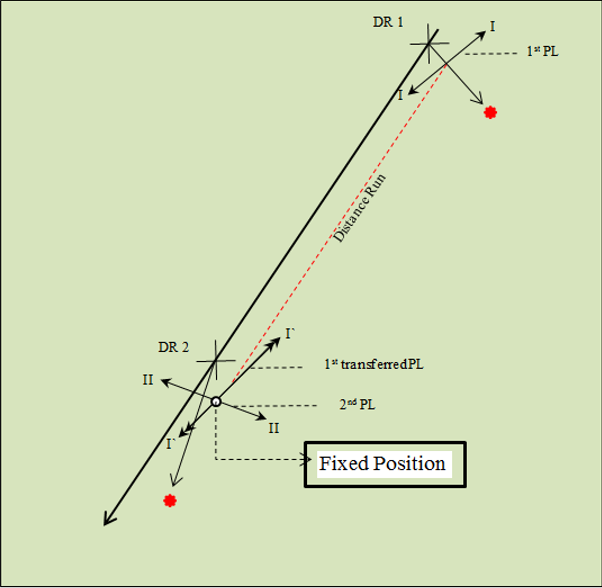### Egyptian Method

In recent years many electronic navigation systems have become out of service Especially those systems that depend on ground stations and determining the location of cruising ships has become dependent on the GPS system Therefore, determining the position using celestial navigation has become of little importance and almost disappears, for several reasons that are summarized as follows:.

• Limited observation time.
• Relatively long calculations for a single astronomical position line.
• Draw several lines for the fixed position, then conduct the analysis process to eliminate errors and obtain the Most Probable Observed Position.
• The impossibility of astronomical observation in case of accumulation of clouds or blurring of the horizon.

On the other hand, we can consider that celestial navigation is characterized by the following:

• The locations of the navigational stars have unlimited accuracy.
• Navigational stars do not cost anything compared to the costs of launching satellite operations, following them up, correcting their trajectories, or re-launching them at the end of their lifespan or failure.
• Celestial navigation can be considered as a supportive (alternative) system for GPS navigation if a personal computer is used to find the Most Probable Observed Position.
##### Using spherical trigonometry to find the Most Probable Observed Position.

The first to present the idea of ​​solving analytically using spherical trigonometry was the Yugoslav Kotlaric in 1954 when he published tables for solving selected pairs of navigational stars in 1971 This method did not succeed for several reasons:

• Practically adhere to the observation of pairs of navigational stars only.
• The tables are not available on a global scale because they are local.

In the current method for the analytical solution using spherical trigonometry which we called the Egyptian method the observer introduce the data of the observed navigational stars (time / sextant altitude) with a maximum of 7 stars as well as the coordinates of these stars extracted from the Nautical Almanac in addition to the traditional data such as the ship's true course, speed and DR position At the end of the solution program, the (Most Probable Observed Position) is obtained.

This procedure was practically applied while sailing (1997) on the training ship Aida 4 (Arab Academy for Science, Technology and Maritime Transport) and compared it with the position obtained from the GPS system, and the results were within the permissible limits

Data to be introduced:

##### Two sets of data are entered;
• The first set is the general data for sailing in addition to the GHA γ which is extracted from the Nautical Almanac tables at the time it is required to find the observed position of the ship.
• The second set is the data for each star.

Detail it as follows.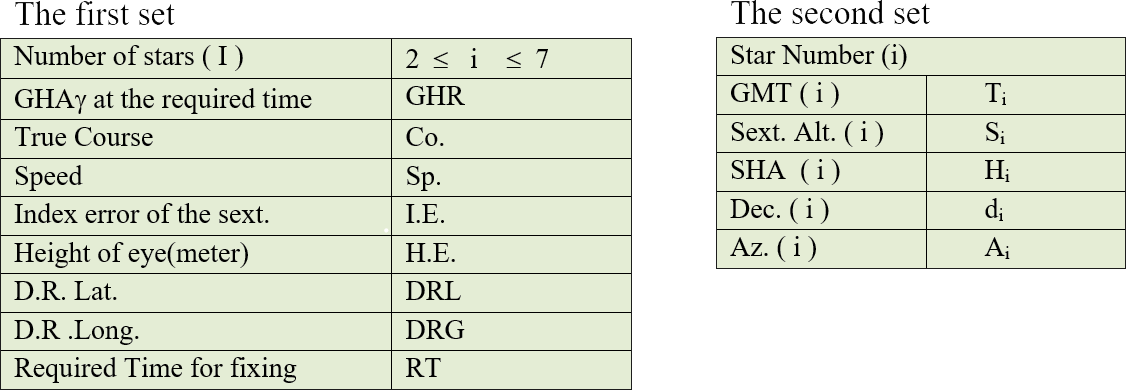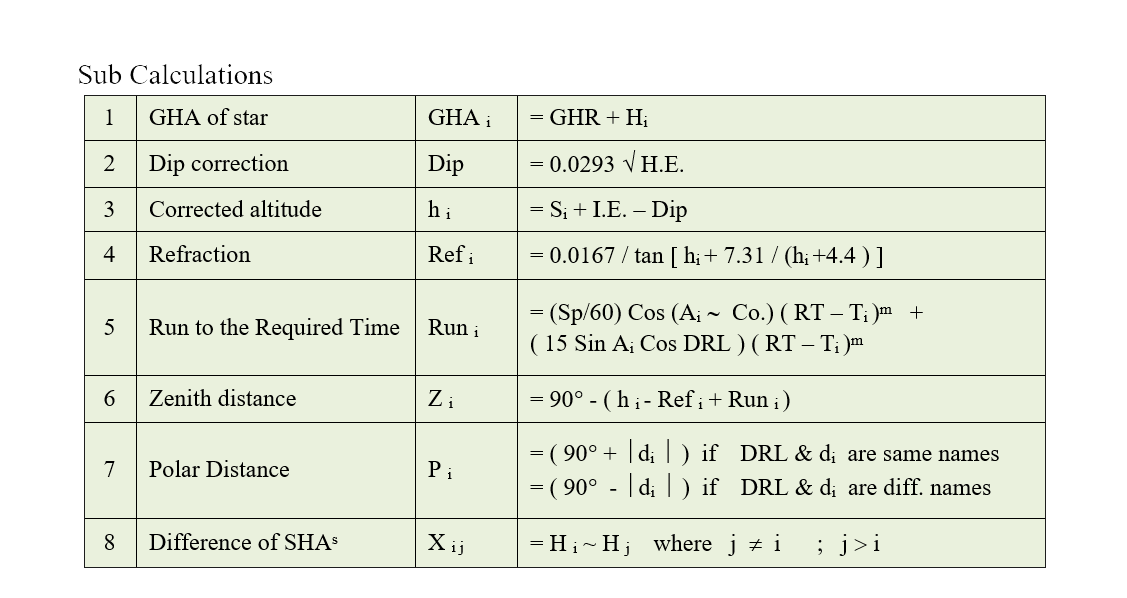Main Calculations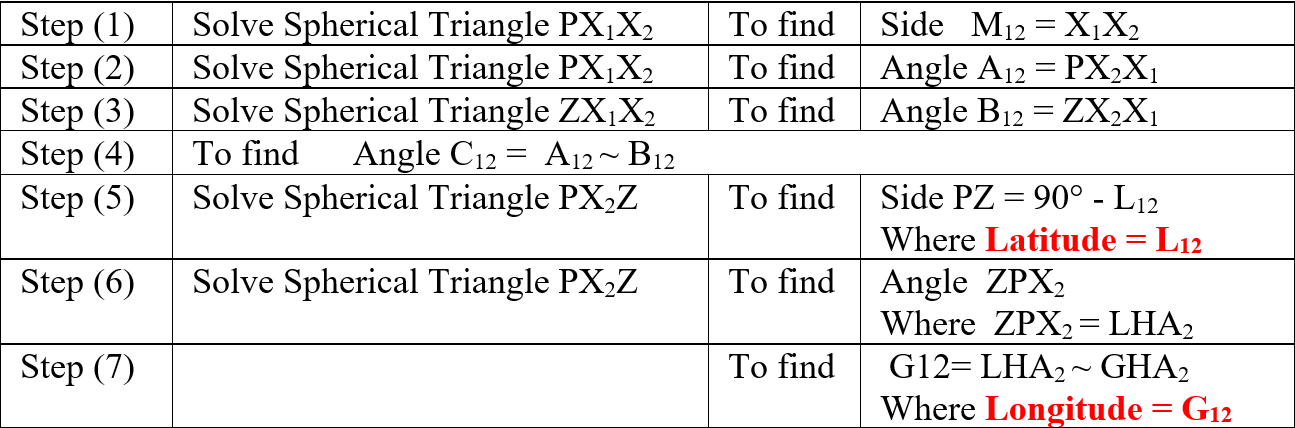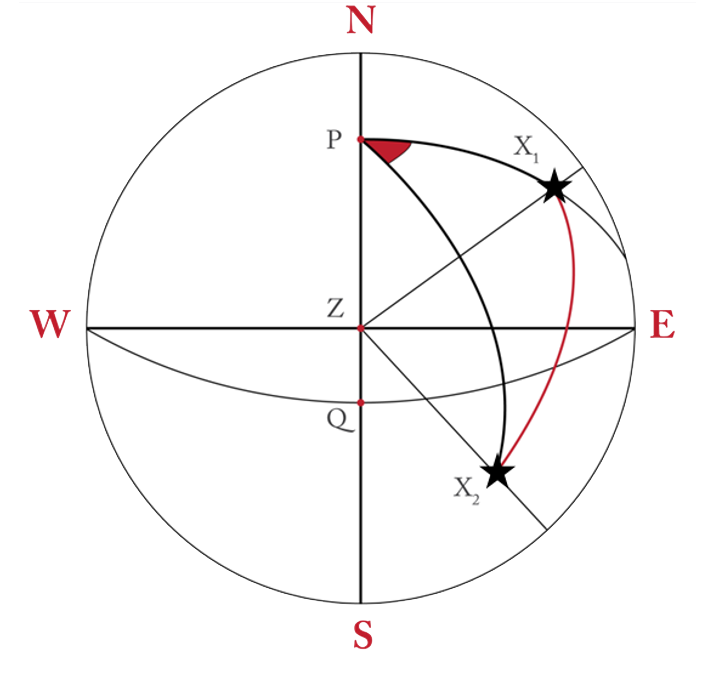##### The above is the solution method for the two stars (1); (2); this is repeated for star pairs (1),(3) and(1),(4) and so on

The following table shows the pairs of stars that are solved; where the maximum number is 21 solutions in the case of observing (7) stars.

But the program is designed so that it eliminates the solution of the pair of stars whose bearings difference is <30° or between (180° ± 30°); Thus, the number of solutions may be < 21.

We assume that the number of pairs of stars (Xi X j) that have been solved is n; to find the MPOP, we complete the solution as follows: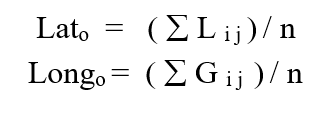Apply it now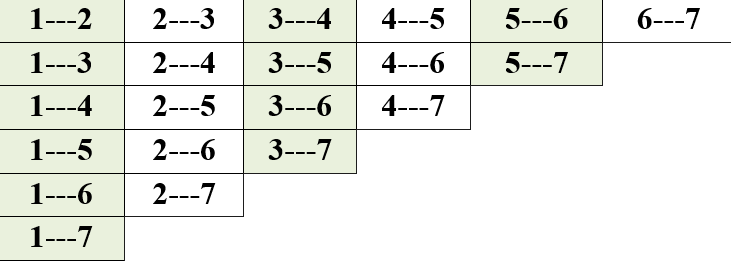### Universal Method

Until the beginning of the era of satellite navigation the most common method for finding the most probable observed position of the ship was the method of French Naval Captain (later a naval general) Marc Saint-Hilaire published in 1875 which was known as the Intercept Method for ease.

This method is summarized in finding the so-called elements of the astronomical position line, which are the Intercept and the True Bearing of the observed celestial body and then the astronomical position line can be drawn i.e. the geometric position of the ship’s position at the time of the observation.

The genius of this method lies in the fact that whatever the error is in the DR position used in the solution we eventually get the same position line on the chart under one condition, which is the plotting of the elements from the DR position used.

It is obvious that in order to find a position, at least two position lines are required to intersect at a point that is the observed position to increase the accuracy several lines of the position are required noting that each of them was monitored at a different times. Therefore, the time of each observation must first be unified for one agreed time.

Then we face the biggest problem which is how to deal with the lot of position lines arising from plotting the lines on the chart or the plotting sheet (the attached figure for illustration).

The current program which I called the Universal Method solves these successive problems especially how to deal with the lot of supposed position lines and then get the Most probable Observed Position at the time required to find the fixed position.

The results were compared with the assumed position (GPS) at the time when the position was required and the difference was not more than (0.7 Mile), which for navigators is a very slight difference during navigation on the high seas.

Apply it now